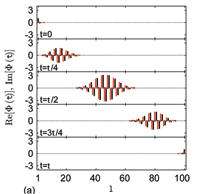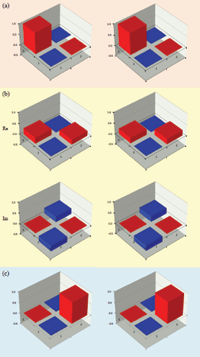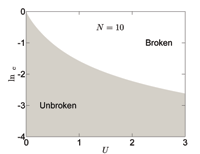June 2011
Mon Tue Wed Thu Fri Sat Sun
« May   Jul »
12345
6789101112
13141516171819
20212223242526
27282930

## Perfect State Transfer in PT-symmetric Non-Hermitian Networks

X. Z. Zhang, L. Jin, Z. SongWe systematically study the parity- and time-reversal- (PT) symmetric non-Hermitian version of a quantum network proposed in the work of Christandl et al. [Phys. Rev. Lett. 92, 187902 (2004)]. We show that such an extended model still allows conditional perfect state transfer within the unbroken PT-symmetric region, but not arbitrary. This is due to the fact that the evolution operator at certain period is equivalent to the PT operator for the real-valued wavefunction in the elaborate PT-symmetric Hilbert space. The critical behavior of the eigenstates is also discussed at the exceptional point.

http://arxiv.org/abs/1106.0087
Quantum Physics (quant-ph)

## Observation of Fast Evolution in Parity-Time-Symmetric System

Chao Zheng, Liang Hao, Gui Lu LongTo find and realize the optimal evolution between two states is significant both in theory and application. In quantum mechanics, the minimal evolution is bounded by the gap between the largest and smallest eigenvalue of the Hamiltonian. In the parity-time-symmetric(PT-symmetric) Hamiltonian theory, it was predicted that the optimized evolution time can be reduced drastically comparing to the bound in the Hermitian case, and can become even zero. In this Letter, we report the experimental observation of the fast evolution of a PT-symmetric Hamiltonian in an nuclear magnetic resonance (NMR) quantum system. The experimental results demonstrate that the PT-symmetric Hamiltonian can indeed evolve much faster than that in a quantum system, and time it takes can be arbitrary close to zero.

http://arxiv.org/abs/1105.6157
Quantum Physics (quant-ph)

## Phase diagram and scaling behavior of a PT symmetric non-Hermitian Bose-Hubbard system

L. Jin, Z. SongWe study the phase diagram of a PT symmetric non-Hermitian Bose-Hubbard model. Using both analytical and numerical approaches, we find that even small on-site interaction can break the PT symmetry drastically. It has been demonstrated that the scaling law can be established for the exceptional point in small U limit. Based on numerical approach, we also find that the phase diagram shows rich structure for medium U: there exist multiple regions in which the PT symmetry is unbroken.

http://arxiv.org/abs/1105.6186
Statistical Mechanics (cond-mat.stat-mech); Quantum Physics (quant-ph)